# Bihar Board 12th Physics Model Question Paper 5 in English Medium

Bihar Board 12th Physics Model Papers

## Bihar Board 12th Physics Model Question Paper 5 in English Medium

Objective Type Questions

There are 1 to 35 objective type questions with 4 options. Choose the correct option which is to be answered on OMR Sheet. (35 x 1 = 35)

Question 1.
The electric field intensity at distance on the axis of an electric dipole is.Ej and E2 on the perpendicular bisector of dipole. The angle between E] and E2 is 6, will be
(a) 1 : 1, π
(b) 1 : 2, π/2
(c) 2 : 1, π
(d) 1 : 3 π
(c) 2 : 1, π

Question 2.
The metre bridge is shown in figure. The value of x is
(a) 10Ω
(b) 3 ohm
(c) 9 ohm
(d) 10 ohm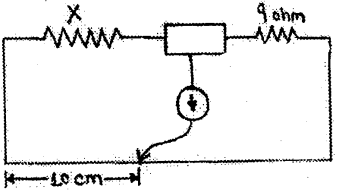(a) 10ΩQuestion 3.
The dimension of electromotive force is
(a) |ML2 T-3]
(b) [ML2T-3I-1]
(c) [MLT-2]
(d) [ML2T-3I-1]
(d) [ML2T-3I-1]

Question 4.
The power of electric circuit is
(a) V.R
(b) V2.R
(c) V2/R
(d) V2RI
(c) V2/R

Question 5.
If a 60W and 40W bulb are joined in series the
(a) 60W bulb glow more
(b) 40 W bulb glow more
(c) Both bulb glow similar
(d) Only 60W bulb is lighted
(b) 40 W bulb glow moreQuestion 6.
Kilowatt hour (kwh) is unit of
(a) Power
(b) Energy
(c) Torque
(d) None of these
(b) Energy

Question 7.
A magnet is cut parallel to its length in n equal
parts. Magnetic moment of each part will be
$$\text { (a) } \frac{M}{n}$$
$$\text { (b) } \frac{M}{n^{2}}$$
$$\text { (c) } \frac{M}{2 n}$$
(d) None of these
$$\text { (a) } \frac{M}{n}$$

Question 8.
The work done to rotate the magnet with 90° will be
(a) MB
(b) MB cosθ
(c) MB sin θ
(d) MB (1 – sin θ)
(b) MB cosθ

Question 9.
S.I. unit of polar strength is
(a) Ampere metre
(b) Tesla
(d) Ampere gm2
(a) Ampere metreQuestion 10.
Which is ferromagnetic substance
(a) Mn
(b) Cr
(c) Zn
(d) Alnico
(d) Alnico

Question 11.
Which law is based on the principle of conservation of energy?
(a) Lenz’s law
(c) Ampere’s law
(d) None of these
(a) Lenz’s law

Question 12.
The unit of reactance is
(a) mho
(b) ohm
(d) ampere
(b) ohmQuestion 13.
The phase difference between current and voltage in AC circuit is 0. Then power factor will
(a) cos θ
(b) sin θ
(c) tan θ
(d) 1θ
(a) cos θ

Question 14.
The velocity of electro magnetic wave in air is(b)

Question 15.
The light waves are transverse in nature which is shown by
(a) Scattering
(b) Diffraction
(c) Interference
(d) Polarisation
(d) PolarisationQuestion 16.
When two converging lens of same total length / are placed in contact then focal length combination is
(a) f
(b) 2f
(c) f2
(d) 3f
(c) f2

Question 17.
When the light ray passes from one medium to another medium then it is deviated, is called
(a) Dispersion
(b) Refraction
(c) Diffraction
(d) Reflection
(b) Refraction

Question 18.
Convex lens are used for
(a) Myopia
(b) Hypermetropia
(c) Old sightedness
(d) Astigmatisn
(b) Hypermetropia

Question 19.
The path difference for destructive interference is
(a) nλ
$$\text { (b) }(2 n+1) \frac{\lambda}{2}$$
(c) Zero
(d) infinity
$$\text { (b) }(2 n+1) \frac{\lambda}{2}$$

Question 20.
Brewler’s law is
(a) μ = sin ip
(b) μ = cos ip
(c) μ = tan ip
(d) μ = tan2 ip
(c) μ = tan ipQuestion 21.
Light is made of vibration by
(a) Ether particle
(b) Air particle
(c) Electric and magnetic field
(d) None of these
(c) Electric and magnetic field

Question 22.
Photo cell is based on
(a) photo electric effect
(b) chemical effect of current
(c) magnetic effect of current
(d) electromagnetic cell
(a) photo electric effect

Question 23.
Which is uncharged
(a) α-particle
(b) β-particle
(c) photon
(d) proton
(c) photon

Question 24.
Lymen series of Hydrogen lies in electromagnetic spectrum is
(a) X : ray
(b) Visible
(c) Infrared
(d) Ultra-violet
(d) Ultra-violetQuestion 25.
When transition takes place in hydrogen atom from higher orbit to another orbit then obtain
(a) Lymen series
(b) Balmer series
(c) Paschan series
(d) Pfund series
(b) Balmer series

Question 26.
The average binding energy per nucleon of nucleus is
(a) 8 ev
(b) 8 Mev
(c) 8 Bev
(d) 8 Joule
(b) 8 Mev

Question 27.
The cause of emission of energy in star is
(a) chemical reaction
(b) fusion of heavy nucleus
(c) fusion of light nucleus
(d) fission of heavy nucleus
(c) fusion of light nucleus

Question 28.
The Boolean expression of OR gate is
(a) A + B = y
(b) A.B = y
$$\text { (c) } \bar{A}=y$$
$$\text { (d) } \mathrm{C}=\overrightarrow{\mathrm{A} \cdot \mathrm{B}}$$
(a) A + B = yQuestion 29.
The permeability of Ferromagnetic substance is
(a) μ > 1
(b) μ = 1
(c) μ < 1
(d) μ = 0
(a) μ > 1

Question 30.
The light is emitted in forward biased junction diode, is called
(a) LED
(b) Photo diode
(c) Zener diode
(d) None of these
(a) LED

Question 31.
Which expression is correct?(d)Question 32.
The majority carriers in n-type semi conductor is
(a) proton
(b) hole
(c) a-particle
(d) electron
(d) electron

Question 33.
Which frequency range is used for TV transmission?
(a) 30 HZ-300HZ
(b) 30 KHZ-300 KHZ
(c) 30 MHZ-300MHZ
(d) 30GHZ-300GHZ
(a) 30 HZ-300HZ

Question 34.
Ionosphere is used for radio waves in
(a) rarer mediun
(b) denser medium
(c) free space
(d) dielectric
(a) rarer mediunQuestion 35.
The maximum distance upto which TV signal can be received from Antenna of height h is proportional to
(a) h1/2
(b) h
(c) h5/2
(d) h2
(a) h1/2

Non-Objective Type Questions!

Question No. 1 to 18 are short answers type question. Each question carries 2 marks. Answer any ten (10) question.

Question 1.
Electric lines of force never intersect to each other. Why?
If two lines of force intersect at a point then it will mean that at that point electric field has two directions. As it is not possible, the lines of force here not intersect to each other.Question 2.
Write the limitations of Coulomb’s law.
Coulomb’s law in electrostatics does not hold in all situation. It is applicable only in following situations :

1. The electric charges must be stationary.
2. The electric charge must be point in size. Coulomb’s law doesn’t apply to two charged bodies of finite size. It is because, the distribution of charge doesn’t remain uniform when the two bodies are brought together.

Question 3.
Prove maximum power theorem.
It states that the output power of a source of emf is maximum, when the external resistance in the circuit is equal to the internal resistance of the source.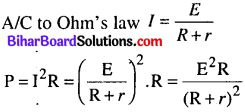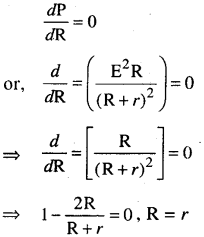This is maximum power theorem.

Question 4.
Establish the relation b/w current density and drift velocity.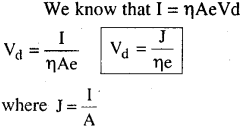Question 5.
What is Hysteresis loop? With its help explain the terms retentivity and co-ercivity.
Hysteresis loop : Hysteresis loop is defined as the lagging of the magnetic induction B behind the corresponding magnetic field H.

When magnetic field H and the magnetic induction B are yarned from zero to maximum in one direction and then back through zero to a maximum in the opposite direction and finally back again through zero to the first maximum, a cycle of magnetisation is said to be completed. B(T) Y Using the value of H and B obtained above, we plot a graph by taking H along x-axis and B along y-axis. When the magnetic field H is increased from zero to the maximum value Og, the induction B follows the curve Oa. However, when the magnetic field is brought to zero, the.;induction does not follows back the curve Oa but a different curve ab. The same pattern of variations is seen for the rest of the curve. The B-H curve for decreasing H does not coincide with the B-H curve for increasing H.As is clear from figure, even when the magnetising field H is zero, some magnetic induction (Ob or Oe) is still present in the speci nen. It is denoted by Br and is called remanance or retent i vity or residual magnetism.

The value of the intensity of magnetisation of a material when the magnetisi ng field is reduced to zero is called retentivity.

After the specimen has been magnetised to saturation (oh or oj) a reversed magnetising field (equal to Oc or Of) is required to reduce the magnetic induction to zero.This is called the coercivity or coercive force He. The value of the magnetising field required to reduce residual magnetism to zero is called coercivity of the material.Question 6.
Write the expression for energy stored in the
The induced emf in coil $$e=-L \frac{d I}{d t}$$
let chasge dq passes from coil, the work done dw = e.dq
\begin{aligned} &=\mathrm{L} \cdot \frac{d I}{d t} d q=\mathrm{L} \cdot d I \cdot \frac{d q}{d t} \\ d w &=\mathrm{LI} . \mathrm{dI} \end{aligned}

work done after posting current O to 1
$$\omega=\mathrm{L} \int_{0}^{1} I d I=\mathrm{L}\left[\frac{I^{2}}{2}\right]_{0}^{1} ; \omega=\frac{1}{2} \mathrm{L}^{2}$$

This work done is stored in the potential energy of coil.
$$P \cdot E=\frac{1}{2} L I^{2}$$

Question 7.
What is current sensitivity and voltage sensitivity of moving coil galvanometer?
Current sensitivity : It is defined as the deflection produced in the galvanometer, when a unit voltage is applied across its cops.
$$\frac{\alpha}{\mathrm{I}}=\frac{\mathrm{nBA}}{\mathrm{K}}$$

Voltage sensitivity : It is defined as the deflection produced in the galvanometer, when a unit voltage is applied across its COPI.
$$\frac{\alpha}{V}=\frac{n B A}{K R}$$

Question 8.
Write the expression for energy density related with electro magnetic wave and show that its ratio is equal to 1.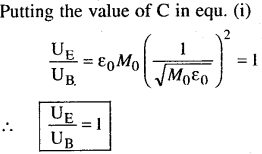Question 9.
Sketch the wave front corresponding to (a)
diverging rays and (b) converging rays.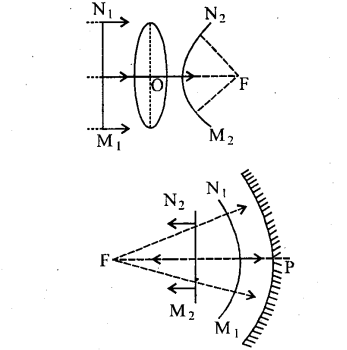Question 10.
Write the differences between magnification and magnifying power.
Magnification :

• It is a linear magnification which is equal to $$\frac{n_{2}}{n_{1}}$$.
• Its value increases with increase in V.
• It may be between to -∞ to +∞.
• Under certain conditions it is equal to magnifying power.Magnifying power :
(i) It is an angular magnification which is equal to $$\frac{\angle \beta}{\angle \alpha}$$
(ii) Its value decreases with increase in V.
(iii) Its value may be b/w $$\frac{d}{f}$$ and $$\left(1+\frac{D}{f}\right)$$
(iv) It is a special condition of magnification when (ve = D

Question 11.
Derive the expression for de-Broglie wave length.
A/C to quantum theory E = hv ……………………….. (i)λ is called de-Broglie wavelength.

Question 12.
What is mass defect?
Mass defect : The difference b/w sum of the masses of neutrons and protons forming a nucleus and actual mass of the nucleus is called mass defect.
Δm = [mpz + mn (A – Z) – M]
The unit of mass defect is amu.Question 13.
Why does thermionic emission takes place from metal surface only?
Thermionic emission takes place from metal surface only because metal has free electrons on its surface.This free electrons requires less energy to come out from one surface. Also sometimes, to reduce work function of metal by coating oxide layer on metal.

Question 14.
Why is semi conductor damaged by a strong current?
A strong current, when passed through a semi conductor, heat up the semi conductor and covalent bond break up. It results in a large number of free electrons. The material behaves as a conductor. As now the semi conductor no longer possesses the property of conduction, it is said to be damaged.

Question 15.
Write the differences between Intrinsic and extrinsic semi conductor.
Intrinsic semi conductor :

• They are the crystals of pure elements like Germanium and Silicon.
• ne = nh
• The electrical conductivity of Intrinsic semi conductor is low.
• Resistivity is higher.Extrinsic semi conductor:

• When some impurity is added in Intrinsic semi conductor, we get an extrinsic semi conductor.
• ne ≠ nh
• Its electrical conductivity is high.
• Resistivity is lower.

Question 16.
Define mean value and root square value A.C. Write its expression.
Mean value of Average value : It is that steady current when passes through the circuit for half time period, sends the same amount of charge of the alternating current, sends the charge in same circuit and in same time period. It is denoted by Im.
\begin{aligned} &I_{m}=\frac{2 I_{0}}{\pi}\\ &I_{m}=0.636 I_{0} \end{aligned}

R.M.S. value or virtual value: It is that steady current when passes through the circuit for half time period, produces the same amount of heat as the alternating current produces the heat in same time and in same circuit. It is denoted by Irms.
\begin{aligned} &I_{r m s}=\frac{I_{0}}{\sqrt{2}}\\ &I_{r m s}=0.7071 \end{aligned}Question 17.
What is polaroids? Write its important uses.
It is a device which produces plane polarised light. Use of polaroids :

• Sun glasses fitted with polaroid sheets protect the eye from glass.
• Wind shields of automobiles are also made of Polaroid sheets.
• They are useful in three dimensional motion pictures.
• It is used in L.C.D., calculator, watches, T.V. etc.

Question 18.
Write the condition for sustained interference.
Condition for sustained interference :

• The two source should emf the light wave continuously.
• The light wave should be of same wave length.
• The two light source should be narrow.
• The light waves emitted should be of the same amplitude.
• The two source of light must lie very close to each other.

Question No. 19 to 24 are long answers type question. Each question of this category carries 5 marks. Answer any three (3) question. (3 x 5 = 15)

Question 19.
Explain any two of the following terms :
(i) Ground Waves
(ii) Sky Waves
(iii) Space Waves.
(i) Ground Waves : The ground waves which progress along the surface of the earth are called ground waves or surface waves. The ground propagation is suitable for low and medium frequency, i.e., up to 2 MHz only, hence it is also called medium wave propagation: The maximum range of ground or surface wave propagation depends on :• The frequency of the radio waves and
• Power of the transmitter.

(ii) The Sky Waves : The sky waves are the radio waves of frequency between 2 MHz to 30 MHz. The ionosphere reflects those radio waves so that they can propagate through atmosphere. The sky waves propagation is also known as ionosphere propagation.

The sky waves are used for very long distance radio communication at medium high frequencies (i.e. at medium waves and short waves). The radio waves can cover a distance approximately 400 km in a single reflection from the ionosphere.

(iii) Space Waves : The space waves are the radio waves of very high frequency (i.e. between 30 MHz to 300 MHz or more). The space waves can travel through atmosphere from transmitter antenna to receive antenna either directly or after reflection from ground in the earth’s troposphere region. It is also called Tropospherical propagation or line of sight propagation. This propagation is limited (a) to the line of sight distance, (b) by the curvature of the earth.

It is utilized in T.V. communication, Radar communication, etc.

Question 20.
Find an expression for the electric field strength at a distant point situated (i) on the axis and (ii) along the equatorial line of an electric dipole.
Consider an electric dipole AB. The charges -q and +q of dipole are situated at A and B respectively. The separation between the charges is 21.

Electric dipole moment, p = Q 21
The direction of dipole moment is from -q to + Q(i) At axial or end-on position : Consider a point P on the axis of dipole at a distance r from mid-point O of electric dipole.The distance of point P from charge + q is BP = r – l and distance of point P from charge – q is, AP = r + l. Let E1 and E2 be the electric field strengths at point P due to charges +<7 and -q respectively. We know that the direction of electric field due to a point charge is away from positive charge and towards the negative charge. Therefore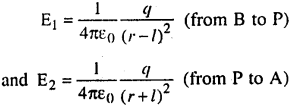Clearly the directions of electric field strengths $$\overrightarrow{\mathrm{E}}_{1}$$ and $$\overrightarrow{\mathrm{E}}_{2}$$ are along the same line but opposite to each other and E1 > E2 because positive charge is nearer.

∴ The resultant electric field due to electric dipole has magnitude equal to the difference of E1 and E2 and direction from 13 to P i.e.If the dipole is infinitely small and point P is far away from the dipole, then r >> l, therefore equation (i) may be expressed as
$$\mathrm{E}=\frac{1}{4 \pi \varepsilon_{0}} \frac{2 p r}{r^{4}} \text { or } \mathrm{E}=\frac{1}{4 \pi \varepsilon_{0}} \frac{2 p}{r^{3}}$$ …………………………… (ii)

This is the expression for the electric field strength at axial position due to a short electric dipole.

Question 21.
Explain the principle and working of a Cyclotron with the help of a neat diagram. Write the expression for Cyclotron frequency.
Or,With the help of a labelled diagram, state the underlying principle of a Cyclotron. Explain clearly how it works to accelerate the charged particles.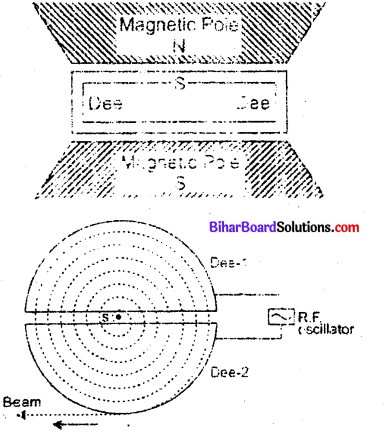Show that Cyclotron frequency is independent of energy of the particle. Is there an upper limit on the energy acquired by the particle? Give reason.
(a) Cyclotron : The cyclotron, devised by Lawrence and Livingston, is a device for accelerating ions to high speed by the repeated application of accelerating potentials.

Construction:
The cyclotron consists of two flat semi-circular metal boxes called ‘dees’ and are arranged with a small gap between them. A source of ions is located near the mid-point of the gap between the dees (fig). The dees are connected to the terminals of a radio frequency oscillator, so that a high frequency alternating potential of several million cycles per second exists between the dees. Thus dees act as electrodes. The dees are enclosed in an insulated metal box containing gas at low pressure. The whole apparatus is placed between the poles of a strong electro¬magnet which provides a magnetic field perpendicular to the plane of the dees.

Working :
The principle of action of the apparatus is shown in fig.The positive ions produced from a source S at the centre are accelerated by a dees which is at negative potential at that moment. Due to the presence of perpendicular magnetic field the ion will move in a circular path inside the dees. The magnetic field and the frequency of the applied voltages are so chosen that as the ion comes out of a dees, the dees change their polarity (positive becoming negative and vice-versa) and the ion is further accelerated and moves with high velocity along a circular path of greater radius. The phenomenon is continued till the ion reaches at the periphery of the deeS where an auxiliary negative electrode (deflecting plate) deflects the accelerated ion on the target to be bombarded.The function of electric field is to accelerae the charged particle and so to impart energy to the charged particle.

The function of magnetic field is to provide circular path to charged particle and so to provide the location where charged particle is capable of gaining energy from electric field.

Expression for Period of Revolution and Frequency :

Suppose the positive ion with charge q moves in a dees with a velocity v, then,
$$q v \mathrm{B}=\frac{m v^{2}}{r} \text { or }=\frac{m v}{q \mathrm{B}}$$ …………………………… (i)

where m is the mass and r is the radius of the path of ion in the dees and B is the strength of the magnetic field. The angular velocity to of the ion is given by.
$$\omega=\frac{v}{r}=\frac{q \mathrm{B}}{m}( \text { from eq. }$$ ………………… (ii)

The time taken by the ion in describing a semi-circle, i.e., in turning through an angle π is.
$$t=\frac{\pi}{\omega}=\frac{\pi m}{\mathrm{B} q}$$

Thus the time is independent of the speed of the ion i.e., although the speed of the ion goes on increasing with increase in the radius (from eQuestion i) when it moves from one dees to the other, yet it take the same time in each dees.

From eQuestion (iii) it is clear that for a particular ion, $$\frac{m}{q}$$

being known, B can be calculated for producing resonance with the high frequency alternating potential.

(b) Resonance Condition : The condition of working of cyclotron is that the frequency of radio frequency alternating potential must be equal to the frequency of revolution of charged particles within the dees. This is called resonance condition.

Now for the cyclotron to work, the applied alternating potential should also have the same semiperiodic time (T/2) as that taken by the ion to cross either dees, i.e.,

This is the expression for period of revolution. Obviously, period of revolution is independent of speed of charged particle and radius of circular path.∴ Frequency of revolution of particles.
$$f=\frac{1}{T}=\frac{q B}{2 \pi m}$$

This frequency is called the cyclotron frequency. Clearly the cyclotron frequency is independent of speed of particle.

(c) Expression for K.E. attained : If R be the radius of the path and Vmax, the velocity of the ion when it leaves the periphery, then in accordance with eq (ii)
$$v_{\max }=\frac{q \mathrm{BR}}{m}$$ …………………………….. (vi)

The kinetic energy of the ion when it leaves the apparatus is,
$$\text { K.E. }=\frac{1}{2} m v^{2} \max =\frac{q^{2} B^{2} R^{2}}{2 m}$$ ………………….. (vii)

When charged particle crosses the gap between dees it gains KE = qV

In one revolution, it crosses the gap twice, therefore if it completes ^-revolutions before emerging the dees, the kinetic energy gained = 2nqV ………………. (viii)
$$\text { Thus, K.E. }=\frac{g^{2} \mathrm{B}^{2} \mathrm{R}^{2}}{2 m}=2 n q \mathrm{V}$$Question 22.
Using Ampere’s circuital law, derive an expression for the magnetic field along the axis of a toroidal solenoid.
Magnetic field due to a toroidal solenoid : A long solenoid shaped in the form of closed ring is called toroidal solenoid (or endless solenoid). Let n be the number of turns per unit of toroid and I the current flowing through it. The current causes the magnetic field inside the turns of the solenoid. The magnetic lines of force inside the toroid are in the form of concentric circle. By symmetry of the magnetic field it has the same magnitude at each point of circle and is along the tangent at every point on the circle.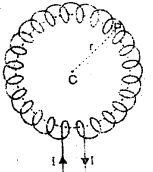For points inside the core of toroid: Consider a circle of radius r in the region enclosed by turns of toroid. Now we apply Ampere’s circuital law to this circular path, i.e.,
$$\vec{f} \overrightarrow{\mathrm{B}} \cdot \overrightarrow{d l}=\mu_{0} I$$ ………………. (i)

$$\vec{f} \overrightarrow{\mathrm{B}} \cdot \overrightarrow{d l}=f B d l \cos 0=B \cdot 2 \pi r$$ Length of toroid = 2πr Number of turns in toroid = n (2πr) and current in one- turn = I.

∴ Current enclosed by circular path = (n 2πr) • 1
∴ Equation (i) gives
$$\mathrm{B} 2 \pi r=\mathrm{m}_{0}(n 2 \pi r l) \Rightarrow \mathrm{B}=\mu_{0} n \mathrm{l}$$Derivation of formula for magnetic field due to a current carrying wire using Biot-Savart law : Consider a wire EF carrying current I in upward direction. The point of observation is P at a finite distance R from the wire. If PM is perpendicular dropped from P on wire; then PM = R. The wire may be supposed to be formed of a large number of small current elements. Consider a small element CD of length δf at a distance f from M.$$\text { Let } \angle \mathrm{CPM}=\phi \text { and } \angle \mathrm{CPD}=\delta \phi, \angle \mathrm{PDM}=\theta$$ The length δf is very small, so that ∠PCM may also be taken as equal to θ.

The perpendicular dropped from C on PD is CN.

The angle formed between element I $$\overrightarrow{\delta l}$$ and $$\overrightarrow{r}$$ (= cp is (π – θ), Therefore according to Biot-Savart law, the magnetic field due to current element I $$\overrightarrow{\delta l}$$ at P is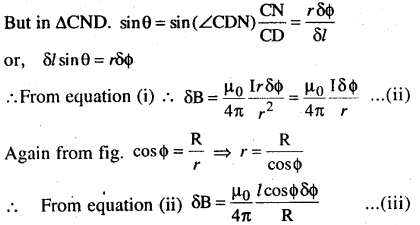If the wire is of finite length and its ends make angles a and p with line MP, then net magnetic field (B) at P is obtained by summing over magnetic fields due to all current elements, i.e.,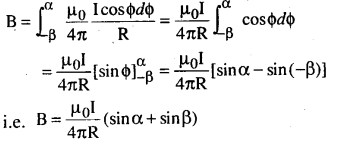This is expression for magnetic field due to current carrying wire of finite length (or very long), then α = β ⇒ π/2.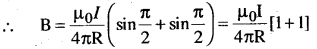Question 23.
Draw the labelled ray diagram for the formation of image by a compound microscope.
Derive an expression for its total magnification (or magnifying power), when the find image is formed at the near point.
Why both objective and eye piece of a compound microscope must have their short focal lengths?
Compound Microscope :
It consists of a long cylindrical tube, containing at one end of a convex lens of small aperture and small focal length. This is called the objective lens (O). At the other end of the tube another co¬axial smaller and wide tube is fitted which carries a convex lens (E) at its outer end. This lens is towards the eye and is called the eye-piece. The focal length and aperture of eye piece are somewhat large than those of objective lens. Cross¬wires are mounted at a definite distance before the eye piece. The entire tube can be moved forward and backward by the rack and pinion arrangement.Magnifying power of a microscope:
is defined as the ratio of angle ((3) subtended by final image on the eye to the angle (a) subtended by the object on eye, when the object is placed at the least distance of distinct vision, i.e.,Magnifying power $$M=\frac{\beta}{\alpha}$$

As object is very small, angles α and β are very small and so tan α = α and tan β = β. By  efinition the object AB is placed at the least distance of distinct vision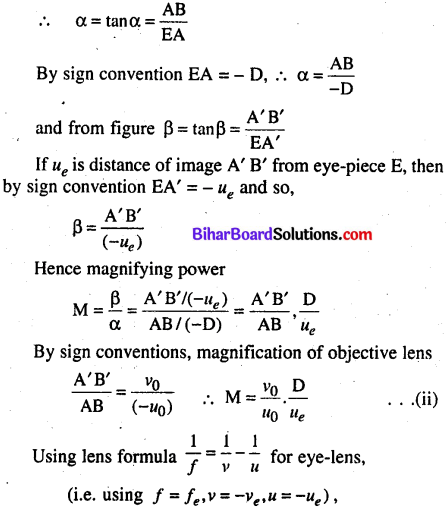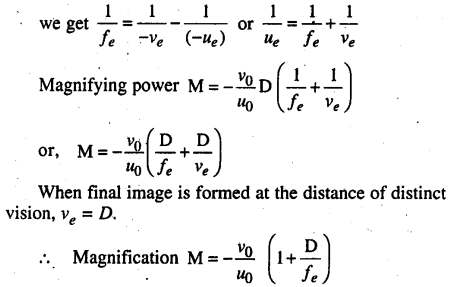Question 24.
State the principle of working of p-n diode as a rectifier. Explain with the help of a circuit diagram, the use of p-n diode as full wave rectifier. Draw a sketch of the input and output wave forms. Or, Draw a circuit diagram of a full wave rectifier. Explain the working principle. Draw the input/output wave forms indicating clearly the functions of the two diodes used.
Rectification :
Rectification means conversion of a.c. into d.c. A p-n diode acts as a rectifier because an ac changes polarity periodically and a p-n diode conducts only when it is forward biased; it does not conduct when reverse biased.In a full wave rectifier, if input frequency is / hertz, then output frequency will be 2/ hertz because for each cycle of input, two positive half cycles of output are obtained.

Working:
The AC input voltage across secondary s1 and s2 cli anges polarity after each half cycle. Suppose during the first half cycle of input AC signal, the terminal s1 is positive relative to centre tap O and s2 is negative relative to O. Then diode D1 is forward biased and diode D2 is reverse biased. Therefore diode D1 conducts while diode D2 does not. The direction of current (i1) due to diode D1 in load rsistance RL is directed from A to 13. In next half cycle, the terminal s is negative and s2 is positive relative to centre tap O.The diode D is reverse biased and diode D2 is forward biased. Therefore, diode D2 conducts while D1 does not. The direction of current (i2) due to diode D2 in load resistance RL is still from A to B. Thus the current in load resistance RL is in the same direction for both half cycles of input AC voltage. Thus for input AC signal the output current is a continuous series of unidirectional pulses.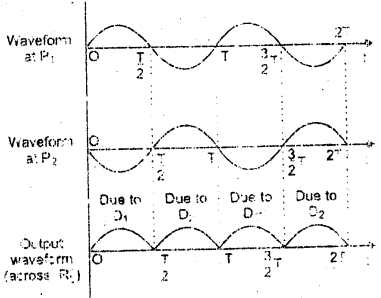In a full wave rectifier, if input frequency is f hertz, then output frequency will be 2f hertz because for each cycle of input, two positive half cycles of output are obtained.error: Content is protected !!